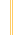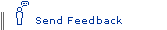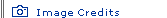# E = mc2 ExplainedEinstein's Big Idea homepage

E = mc2. It's the world's most famous equation, but what does it really mean? "Energy equals mass times the speed of light squared." On the most basic level, the equation says that energy and mass (matter) are interchangeable; they are different forms of the same thing. Under the right conditions, energy can become mass, and vice versa. We humans don't see them that way—how can a beam of light and a walnut, say, be different forms of the same thing?—but Nature does.

So why would you have to multiply the mass of that walnut by the speed of light to determine how much energy is bound up inside it? The reason is that whenever you convert part of a walnut or any other piece of matter to pure energy, the resulting energy is by definition moving at the speed of light. Pure energy is electromagnetic radiation—whether light or X-rays or whatever—and electromagnetic radiation travels at a constant speed of 300,000 km/sec (186,000 miles/sec).

Why, then, do you have to square the speed of light? It has to do with the nature of energy. When something is moving four times as fast as something else, it doesn't have four times the energy but rather 16 times the energy—in other words, that figure is squared. So the speed of light squared is the conversion factor that decides just how much energy lies within a walnut or any other chunk of matter. And because the speed of light squared is a huge number—90,000,000,000 (km/sec)2—the amount of energy bound up into even the smallest mass is truly mind-boggling.

Here's an example. If you could turn every one of the atoms in a paper clip into pure energy—leaving no mass whatsoever—the paper clip would yield 18 kilotons of TNT. That's roughly the size of the bomb that destroyed Hiroshima in 1945. On Earth, however, there is no practical way to convert a paper clip or any other object entirely to energy. It would require temperatures and pressures greater than those at the core of our sun.Now Check This Out! E = mc2: A Biography of the World's Most Famous Equation by David Bodanis. Berkley Books, 2000. Explore the innovative thinkers behind each piece of the equation, its synthesis by Einstein, and its impact on society. Einstein 1905: The Standard of Greatness by John S. Rigden. Harvard University Press, 2005. Examine the impact of Einstein's work during 1905—the "miraculous year" when he published E = mc2 and four other universe-changing papers. NOVA—Einstein's Big Idea www.pbs.org/nova/einstein Get to know Einstein and his ideas through a time line of significant events in his life, interactive simulations of the speed of light and the effect of motion on time, and essays explaining E = mc2 and Einstein's other contributions.